# 经典sql语句 行专列 统计部门男女人数 统计员工入职时常 根据出生日期计算年龄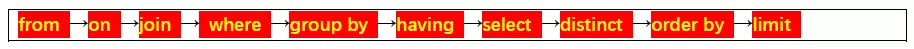1，SELECT ,COUNT() FROM dept d LEFT JOIN emp e ON d.deptno=e.deptno WHERE e.sal<4000 GROUP BY d.deptno

2，

create table tb(姓名 varchar(10),课程 varchar(10),分数 int)
insert into tb values(‘张三’,‘语文’,74)
insert into tb values(‘张三’,‘数学’,83)
insert into tb values(‘张三’,‘物理’,93)
insert into tb values(‘李四’,‘语文’,74)
insert into tb values(‘李四’,‘数学’,84)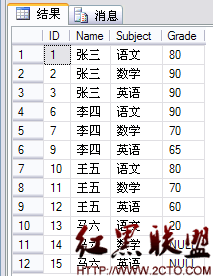SELECT 姓名,SUM(IF(课程=“语文”,分数,0)) AS 语文,
SUM(IF(课程=“数学”,分数,0)) AS 数学,
SUM(IF(课程=“物理”,分数,0)) AS 物理 FROM tb GROUP BY 姓名

– 使用case when （SQL2000以上）
select 姓名,
max(case 课程 when ‘语文’ then 分数 else 0 end)语文,
max(case 课程 when '数学’then 分数 else 0 end)数学,
max(case 课程 when '物理’then 分数 else 0 end)物理
from tb
group by 姓名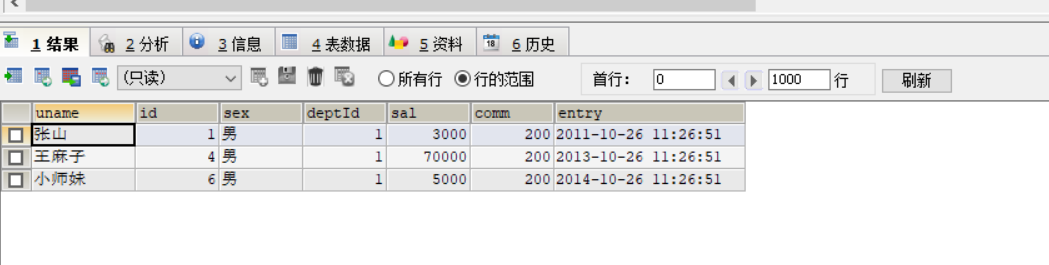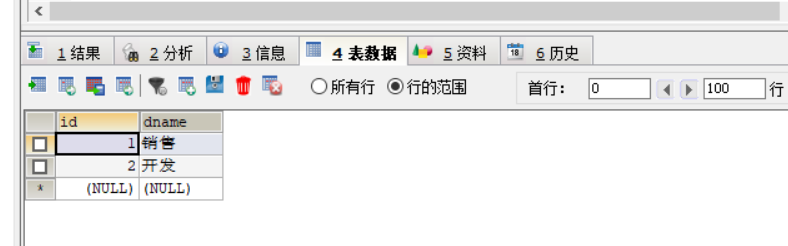SELECT * FROM COM WHERE entry<=DATE_SUB(SYSDATE(),INTERVAL 5 YEAR);

SELECT d.dname,
SUM(CASE WHEN sex = ‘女’ THEN 1 ELSE 0 END) 女,
SUM(CASE WHEN sex = ‘男’ THEN 1 ELSE 0 END) 男 FROM com c LEFT JOIN dept d ON d.id = c.deptId GROUP BY deptId

SELECT d.id,d.dname,c.sex,COUNT(*) FROM com c ,dept d WHERE d.id=c.deptId GROUP BY c.deptId , c.sex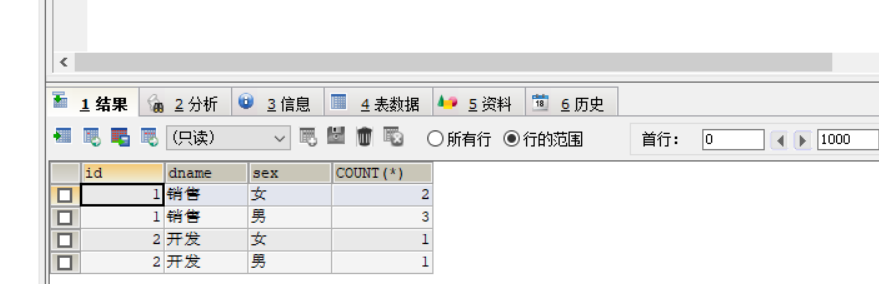CASE WHEN sex = ‘女’ THEN 1 ELSE 0 END
CASE 课程 when ‘语文’ then 分数 else 0 end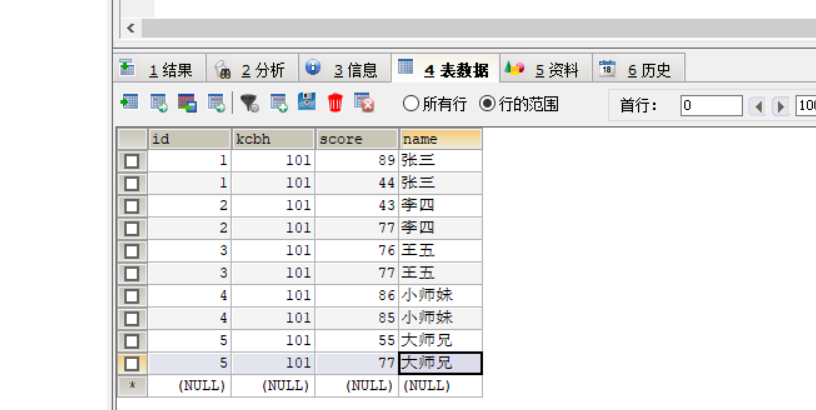方法1：SELECT DISTINCT(NAME) FROM sc WHERE id NOT IN (SELECT DISTINCT(id) FROM sc WHERE score <60)

SELECT NAME FROM sc GROUP BY NAME HAVING MIN(score)>60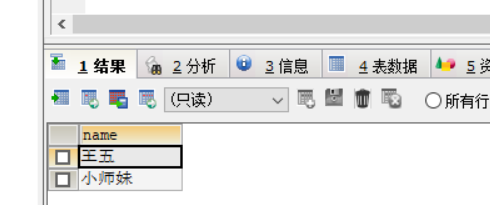SELECT *,TIMESTAMPDIFF(YEAR,entry,CURDATE())AS 年龄 FROM com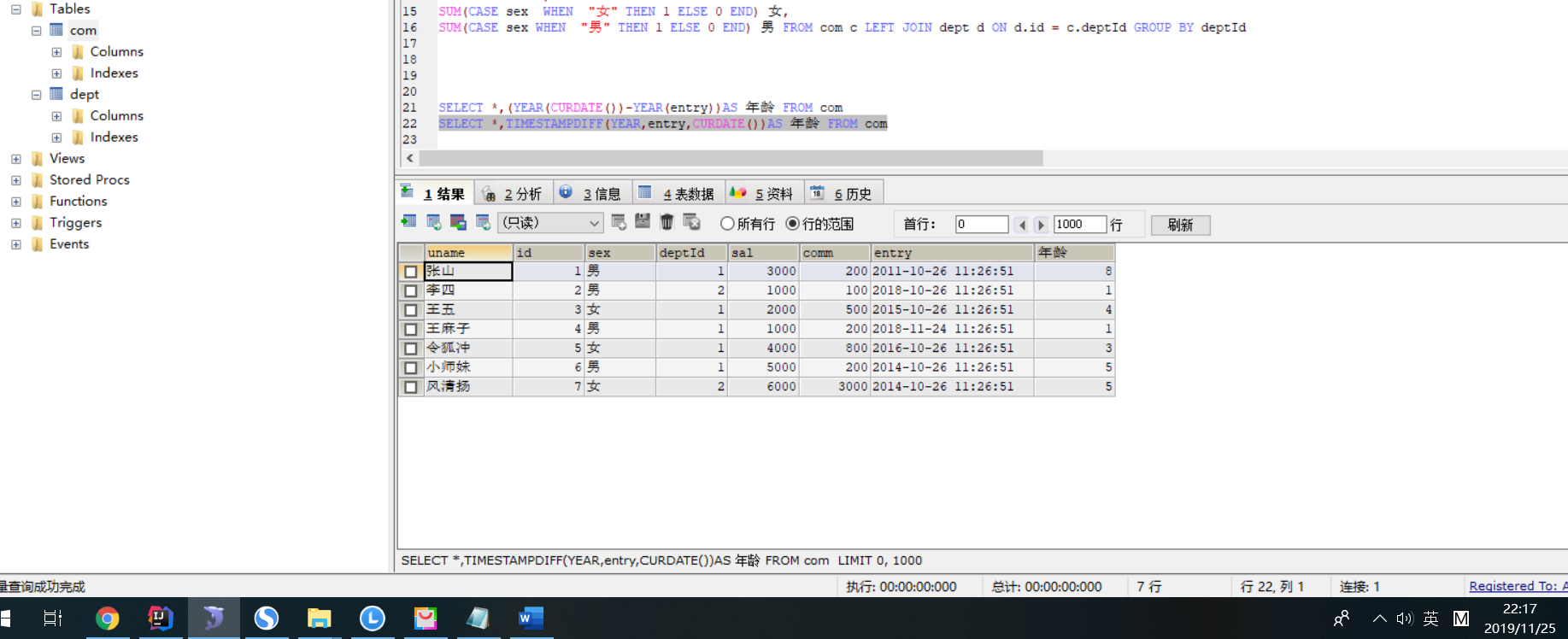©️2019 CSDN 皮肤主题: 大白 设计师: CSDN官方博客